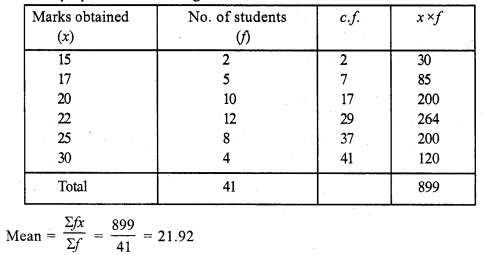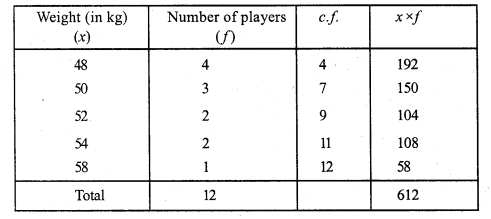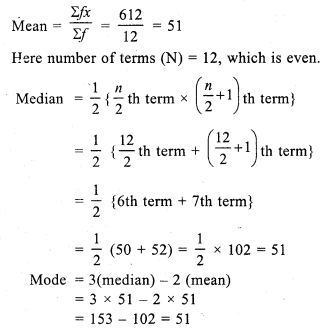## RS Aggarwal Class 7 Solutions Chapter 21 Collection and Organisation of Data (Mean, Median and Mode) Ex 21C

These Solutions are part of RS Aggarwal Solutions Class 7. Here we have given RS Aggarwal Solutions Class 7 Chapter 21 Collection and Organisation of Data Ex 21C.

Other Exercises

Question 1.
Solution:
(i) Arranging in ascending order :
4, 6, 7, 8, 8, 8, 8, 10, 11, 15
We see that 8 occurs maximum times
Mode = 8
(ii) Arranging in ascending order :
18, 21, 23, 27, 27, 27, 27, 27, 36, 39, 40
We see that 27 occurs maximum times
Mode = 27

Question 2.
Solution:
Arranging in ascending order :
28, 31, 32, 32, 32, 32, 34, 36, 38, 40, 41.
We see that 32 occurs maximum times
Mode = 32 years

Question 3.
Solution:
We prepare the table as given below:Here, number of terms = 45, which is odd
Median = $$\frac { n + 1 }{ 2 }$$ th term = $$\frac { 45 + 1 }{ 2 }$$ = $$\frac { 46 }{ 2 }$$ th term
= 23th term = 450
Now, mode = 3(median) – 2(mean)
= 3 x 450 – 2 x 470
= 1350 – 940
= 410

Question 4.
Solution:
We prepare the table as given below:Here, number of terms (N) = 41, which is odd
Median = $$\frac { n + 1 }{ 2 }$$ th term = $$\frac { 41 + 1 }{ 2 }$$ th term
= $$\frac { 42 }{ 2 }$$ = 21 th term = 22 {value of 18 to 29 = 22}
Mode = 3 (median) – 2 (mean)
= 3 x 22 – 2 x 21.92 = 66 – 43.84 = 22.16

Question 5.
Solution:
We prepare the table as given below:Hope given RS Aggarwal Solutions Class 7 Chapter 21 Collection and Organisation of Data Ex 21C are helpful to complete your math homework.

If you have any doubts, please comment below. Learn Insta try to provide online math tutoring for you.